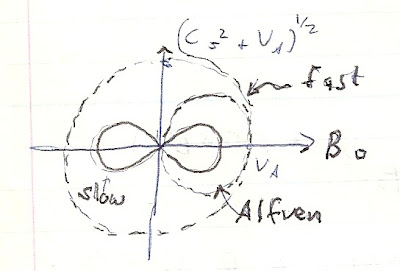## Saturday, April 16, 2011

### Getting a Handle on Alfven Waves (2)We left off trying to obtain the x, y-components of velocity associated with the wave, by use of Fourier transforms. The x-component is:

w v x   –  c s     k    v x  + B  z    k m  r o   [v  z   B  x  –  v  x  B  z] = 0

The y-component is decoupled from the others (x, z) and can be written:

w v y   -  B  x 2   k  v y m o   r o = 0

or simply:

w  = [B  x 2   m o  r o] k 2

where the quantity in brackets is the Alfven velocity or alternatively written:

v  A  = [ w/ k] =  B x  / [m o  r o1/ 2

or

v   = Bo  / [m o  r o1/ 2

since Bo    is in the x –direction

:

For completeness, we should be able to show the z-component equation is:

w v  -  B x  k m o  r o [v  z   B  x –  v  x  B  z] = 0

Here, let me back up and refer readers again to the basic wave equation one can obtain by getting the 2nd derivative of:

v l / t  = -(c s  2 ) grad  p  l  / r  - 1/ m  r  [B o X Curl B 1]

One can then find the solution in terms of plane waves by assuming:

v  1 = v  1 *[exp ik.x – iwt]

for which taking the second derivative, of  v  1 with respect to t yields the original equation in w we found earlier. All of this the energetic reader should be able to work out, but most of it (after taking derivatives) reduces to brute force algebra!

For completeness, I need to note what happens when you solve the preceding (simultaneous) equations in x, and z.

w 4 +  w2 [- c s  2  k   - B x  k  / m o  r o ] + c s  2  k  4    [B x / m o  r o ] = 0

or:

w 4 -   w(c s    + v  A 2 )k  +  c s  2 v  A cos  2 (Θ) k = 0

and finally,

w = ½[( c s  2 v  A k 2  +   [(c s  2 v  A k  4  –  4 c s  2 v  A 2    cos 2 (Θ) k4 ]1/2

Now, if one plots the preceding using for the vertical axis (c s    + v  A 2 ) and for the horizontal B o (e.g. x) one will get what is called “Friedrich’s diagram” (see sketch image). It consists of

1)A smaller “dumb bell” or figure-8 shaped graph centered at the origin. This will be for what we call “slow mode” waves

2)A single larger lobe that envelopes the smaller right lobe of the dumb bell. This will be for Alfven waves proper.

3) A circle shaped graph surrounding both 1, 2 above. This will be for what we call the “fast MHD” mode.

The critical thing to note here is that the fast mode is the only MHD wave able to carry energy perpendicular to the magnetic field. This has important ramifications for solar flares, as well as magnetospheric effects (such as the aurora). Meanwhile, the phase velocity (w /k) of the slow mode wave perpendicular to the magnetic field is always zero. In the limit where the sound speed c s  2  < < v  A 2, and the Alfven speed v  A 2 << c s  2, the slow wave disappears. (Which one can easily validate and confirm for the equation in w)

Other properties, points to note:

-the velocity perturbation v 1 is orthogonal to B o

-the wave is incompressible since DIV v 1 = ik.v 1 = 0

-the magnetic field perturbation (B 1 ) is aligned with the velocity perturbation. Since both are perpendicular to k and B o

-the current density perturbation (J 1) exists as a current perturbation perpendicular to k and B o  e.g.

1 = k X  B o

- When c s  2  <<  v  A 2 the fast mode wave becomes a compressional Alfven wave. This has a group velocity equal to its phase velocity w /k